# append()

## T.append( cs )

 T.append(cs)

T是复组表时，cs可以为单路或多路，单路cs或多路cs的每一路只能对应一个分区，必要时将自动产生缺失的组文件成员，且只能在组文件定义的分区号内产生；T是集群组表时，只能追加集群游标，但当集群组表中已有数据时，可以追加普通游标，此时普通游标可能多路。记录追加以后组表会自动更新索引。

cs是集群分布游标时，必须保证cs与集群组表T的数据结构一致，根据分布表达式写入到集群表相应分区；附表要按照主表的数据执行分段等操作，所以主表中已有对键有序且唯一的数据时，附表才能添加记录。

 T 实表/复组表/集群组表 cs 游标/集群分布游标

 @m 以归并方式追加，不支持附表 @i 立即写出；缺省积累足够数据后再写出，程序退出/有读取时将立即写出 @a 归并追加到补文件上，没有补文件则创建 @x cs为单路游标时，可能对应复组表T的多个分区，需要每次计算分区表达式

Ø  T为实表：

 A 1 =create(k1,v1).record([1,10,2,20,3,30,4,40,10,100]) 创建序表2 =create(k1,k2,v2).record([1,1,"a",3,1,"c"]) 创建序表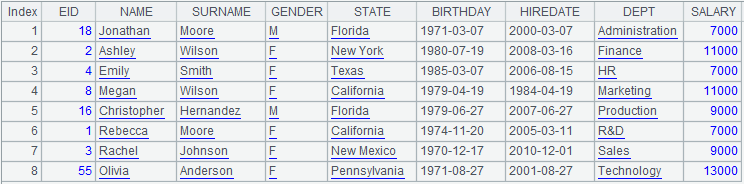3 =create(k1,v3).record([1,"red",1,"blue",2,"red",2, "yellow",2,"red",2,"red",4,"black",4,"red",4,"red", 4,"red",4,"red",4,"red",10,"red"]) 创建序表4 =file("D:/ctb.ctx") 5 =A4.create(#k1,v1) 创建组表基表，其中k1作为维，v1为基表的列 6 =A5.attach(table2,#k2,v2) 在A2返回的基表上增加名为table2的附表，附表的维是k1和k2，列为v2 7 =A5.attach(table3,v3) 在A2返回的基表上增加名为table3的附表，附表的维是k1，列为v3 8 =A5.append(A1.cursor()) 在基表中添加游标记录 9 =A6.append(A2.cursor()) 在附表table2中添加游标记录 10 =A7.append(A3.cursor()) 在附表table3中添加游标记录 11 =A5.cursor().fetch() 返回基表中的数据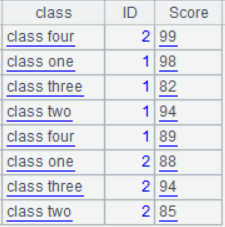12 =A6.cursor().fetch() 返回附表table2中的数据13 =A7.cursor().fetch() 返回附表table3中的数据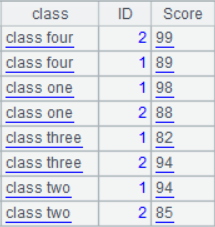14 =demo.cursor("select EID,NAME,GENDER from employee where EID<30") 15 =file("empTest.ctx") 16 =A15.create(#EID,NAME,GENDER) 17 =A16.append@a(A14) 使用@a选项，将A14中的数据归并追加到补文件上，没有则创建补文件empTest_SF.ctxØ  T为复组表：

 A 1 =file("deptTest.ctx":[1,2,3]) 返回组文件 2 =A1.create(#DEPTID,DEPTNAME;;DEPTID%2+1) 生成复组表 3 =demo.cursor("select * from dept") 4 =A2.append@x(A3) A3对应A2的多个分区，需要每次计算分区表达式DEPTID%2+1，将单路游标A3依次追加到复组表中；分区1/2内没有deptTest.ctx，则自动产生缺失的组文件

Ø  T为集群组表：

 A 1 =file("emp.ctx":,["192.168.0.104:8281","192.168.190.129:8281"]) 打开集群文件 2 =A1.open() 返回集群表 3 =file("emp.ctx":,["192.168.0.104:8281","192.168.190.129:8281"]).open().cursor() 返回集群游标 4 =A2.append(A3) 集群组表追加集群游标 5 =file("emp1.ctx":,["192.168.0.104:8281"]) emp1.ctx中有数据 6 =A4.open() 7 =file("d://emp.ctx").open().cursor() 打开本地文件并返回普通游标 8 =A5.append(A6) 集群组表中有数据，可以追加普通游标 9 =A5.append@m(A6) 加上@m选项，以归并方式追加

## f .append()

合并结构相同的两个组表

 f.append( f’,p)

 f’ 组表文件 p 组表文件f的可写密码

 @x 合并完成后删除f’

 A 1 =file("D:\\append1.ctx") 2 =A1.create(#EID,NAME,DEPT,SALARY) 创建组表基表 3 =demo.cursor("select EID,NAME,DEPT,SALARY from EMPLOYEE where EID<10 order by  EID") 4 =A2.append@i(A3) 在实表中添加游标记录，并立即写出5 =file("D:\\append2.ctx") 6 =A5.create(#EID,NAME,DEPT,SALARY) 创建组表基表 7 =demo.cursor("select EID,NAME,DEPT,SALARY from EMPLOYEE where  EID>10and  EID<20  order by  EID") 8 =A6.append@i(A7) 在实表中添加游标记录，并立即写出9 =A1.append(A5) 将不加密组表f’合并到不加密组表f后 10 =A9. open ().cursor().fetch() 合并后组表数据内容如下：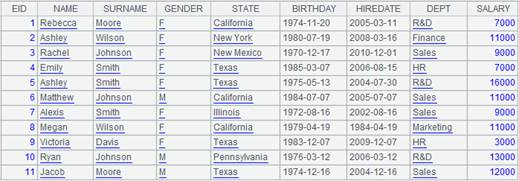11 =file("D:\\append3.ctx") 12 =A11.create(#EID,NAME,DEPT,SALARY;;;"123":"11") 创建加密的组表基表，写入密码为123，读取密码为11 13 =demo.cursor("select EID,NAME,DEPT,SALARY from EMPLOYEE where EID<5 order by  EID") 14 =A12.append@i(A13) 在实表中添加游标记录，并立即写出15 =A11.append(A5,"123") 将不加密组表f’合并到加密组表f后 16 =A11.open("11").cursor().fetch()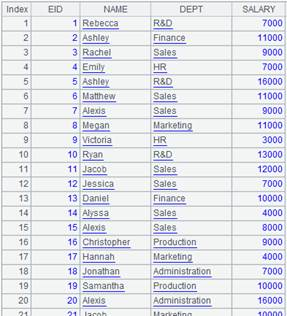17 =A1.append@x(A5) 使用@x选项合并两组表，合并后删除A5中的组表append2.ctx